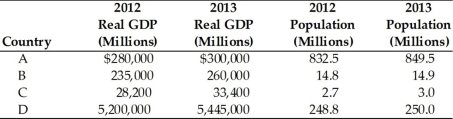• FAQ
• Contact/ Homework Answers / Economics / 9.1  How Do We Define Economic Growth? 1) An increase in
Not my Question
Flag Content

# Question

9.1  How Do We Define Economic Growth?

1) An increase in growth rates will cause the production possibilities curve to

A) shift inward.

B) become steeper.

C) become flatter.

D) shift outward.

2) An outward shift of the production possibilities curve represents

A) economic contraction.

B) economic growth.

C) economic recession.

D) economic inflation.

3) Which of the following variables is used to measure economic growth?

A) nominal GDP

B) nominal GDP per capita

C) real GDP

D) real GDP per capita

4) According to your text, which of the following countries currently has the highest annual per capita Gross Domestic Product (GDP) growth rate?

A) China

B) Japan

C) Germany

D) United States

5) The term "economic" growth refers to increases in

A) resources use.

B) nominal income.

C) satisfaction.

D) productive capacity.

6) Economic growth is usually defined as

A) the rate of increase in output divided by the increase in labor.

B) the increase in output over time, as measured by real per capita Gross Domestic Product (GDP).

C) the increase in input availability.

D) the reduction in the real cost of necessities.

7) Using a production possibilities curve, economic growth is represented by

A) an outward shift in the curve.

B) a movement along the curve.

C) an inward shift of the curve.

D) a pivot of the curve.

8) Economic growth is best measured by increases in

A) nominal personal income.

B) nominal Gross Domestic Product (GDP).

C) per capita real Gross Domestic Product (GDP).

D) per capita nominal Gross Domestic Product (GDP).

9) When Country X has high economic growth, this country has

A) a high level of real Gross Domestic Product (GDP).

B) a high level of per capita real Gross Domestic Product (GDP).

C) a large increase in per capita real Gross Domestic Product (GDP).

D) a large increase in personal income.10) Refer to the above table. Which country experienced the greatest economic growth from 2012 to 2013?

A) A

B) B

C) C

D) D

## Solution 5 (1 Ratings )

Solved
Economics 7 Months Ago 23 Views